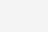python格式化经纬度的方法1、对数据进行清理，主要是度分秒的符号问题，有的是中文或者英文，需要统一替换成一种在Excel中完成。

2、把度分秒的数字提取出来，分别处理。

3、格式化使用format函数。

import pandas as pd
import re

df = pd.read_excel('1.xlsx', sheet_name = 'Sheet1')

def get(x):
degree = re.findall('(\d+)°', x)
minute = re.findall('° (\d+)', x)
second = re.findall('''' (.*?)"''', x)
return '''{}°{:02d}'{:05.2f}" N'''.format(degree, int(minute), float(second))
df['lat'] = df['纬度'].apply(get)
df